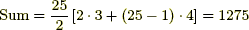index: click on a letter A B C D E F G H I J K L M N O P Q R S T U V W X Y Z A to Z index index: subject areas numbers & symbols sets, logic, proofs geometry algebra trigonometry advanced algebra & pre-calculus calculus advanced topics probability & statistics real world applications multimedia entrieswww.mathwords.com about mathwords website feedback

Arithmetic Series

A series such as 3 + 7 + 11 + 15 + ··· + 99 or 10 + 20 + 30 + ··· + 1000 which has a constant difference between terms. The first term is a1, the common difference is d, and the number of terms is n. The sum of an arithmetic series is found by multiplying the number of terms times the average of the first and last terms.

 Formula:orExample: 3 + 7 + 11 + 15 + ··· + 99 has a1 = 3 and d = 4. To find n, use the explicit formula for an arithmetic sequence. We solve 3 + (n – 1)·4 = 99 to get n = 25.or